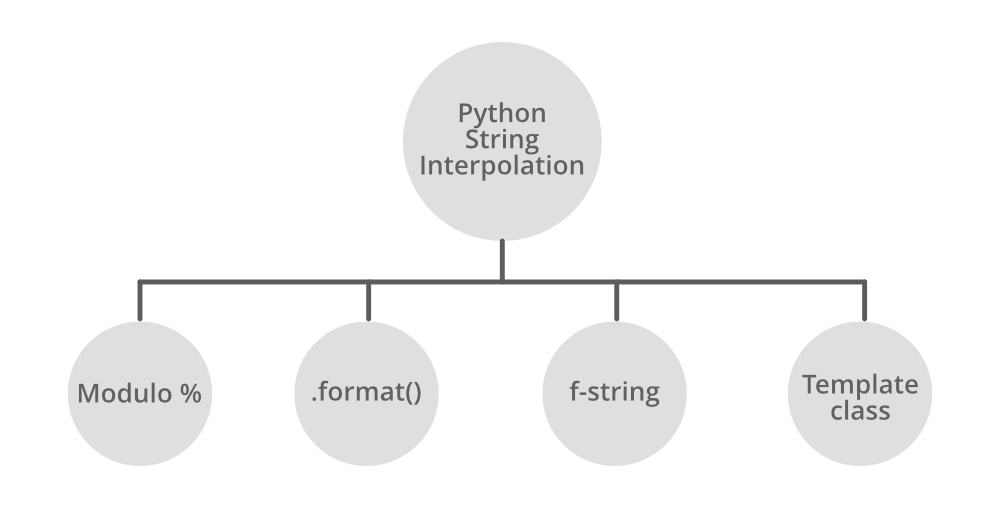GFG App
Open AppBrowser
Continue

# Python String Interpolation

String Interpolation is the process of substituting values of variables into placeholders in a string. Let’s consider an example to understand it better, suppose you want to change the value of the string every time you print the string like you want to print “hello <name> welcome to geeksforgeeks” where the <name> is the placeholder for the name of the user. Instead of creating a new string every time, string interpolation in Python can help you to dynamically change the placeholder with the name of the user.## % – Formatting

% – Formatting is a feature provided by Python which can be accessed with a % operator. This is similar to printf style function in C.

Example: Formatting string using % operator

## Python3

 `# Python program to demonstrate` `# string interpolation`     `n1 ``=` `'Hello'` `n2 ``=` `'GeeksforGeeks'`   `# for single substitution` `print``(``"Welcome to % s"` `%` `n2)`   `# for single and multiple substitutions()` `# mandatory` `print``(``"% s ! This is % s."` `%` `(n1, n2))`

Output

```Welcome to GeeksforGeeks
Hello ! This is GeeksforGeeks.```

Let’s say it’s just a complicated version, but we can use it if we have a lot of variables to get substituted in the string as we don’t always want to use(“string” + variable + “string” + variable + variable + “string”) this representation. So for this purpose, we can go with %-formatting.

Note: To know more about %-formatting, refer to String Formatting in Python using %

## Str.format()

str.format()work by putting in one or more replacement fields and placeholders defined by a pair of curly braces { } into a string. The value we wish to put into the placeholders and concatenate with the string passed as parameters into the format function.

Example: Formatting strings using format() method

## Python3

 `# Python program to demonstrate` `# string interpolation`     `n1 ``=` `'Hello'` `n2 ``=` `'GeeksforGeeks'`   `# for single substitution` `print``(``'{}, {}'``.``format``(n1, n2))`

Output

`Hello, GeeksforGeeks`

We can also use the variable name inside the curly braces {}. This will allow us to use the parameters of format functions in any order we want.

Example: Format functions with variables inside curly braces.

## Python3

 `n1 ``=` `"Hello"` `n2 ``=` `"GeeksForGeeks"`   `# for single or multiple substitutions` `# let's say b1 and b2 are formal parameters` `# and n1 and n2 are actual parameters` `print``(``"{b1}! This is {b2}."``.``format``(b1``=``n1, b2``=``n2))`   `# we can also change the order of the ` `# variables in the string without changing ` `# the parameters of format function` `print``(``"{b2}! This is {b1}."``.``format``(b1``=``n1, b2``=``n2))`

Output

```Hello! This is GeeksForGeeks.
GeeksForGeeks! This is Hello.```

Note: To know more about str.format(), refer to format() function in Python

## f-strings

PEP 498 introduced a new string formatting mechanism known as Literal String Interpolation or more commonly as F-strings (because of the leading f character preceding the string literal). The idea behind f-strings is to make string interpolation simpler.

To create an f-string, prefix the string with the letter “ f ”. The string itself can be formatted in much the same way that you would with str.format(). F-strings provide a concise and convenient way to embed python expressions inside string literals for formatting.

Example: Formatting Strings using f-strings

## Python3

 `# Python program to demonstrate` `# string interpolation`     `n1 ``=` `'Hello'` `n2 ``=` `'GeeksforGeeks'`   `# f tells Python to restore the value of two` `# string variable name and program inside braces {}` `print``(f``"{n1}! This is {n2}"``)`

Output

```Hello! This is GeeksforGeeks
(2 * 3)-10 = -4```

We can also use f-strings to calculate some arithmetic operations and it will perform the inline arithmetic. See the below example –

Example: Inline arithmetic using f-strings

## Python3

 `a ``=` `2` `b ``=` `3` `c ``=` `10`   `print``(f``"({a} * {b})-{c} = {(2 * 3)-10}"``)`

Output

`(2 * 3)-10 = -4`

Note: To know more about f-strings, refer to f-strings in Python

## String Template Class

In the String module, Template Class allows us to create simplified syntax for output specification. The format uses placeholder names formed by \$ with valid Python identifiers (alphanumeric characters and underscores). Surrounding the placeholder with braces allows it to be followed by more alphanumeric letters with no intervening spaces. Writing \$\$ creates a single escaped \$:

Example: Formatting string using Template Class

## Python3

 `# Python program to demonstrate` `# string interpolation`     `from` `string ``import` `Template`   `n1 ``=` `'Hello'` `n2 ``=` `'GeeksforGeeks'`   `# made a template which we used to` `# pass two variable so n3 and n4` `# formal and n1 and n2 actual` `n ``=` `Template(``'\$n3 ! This is \$n4.'``)`   `# and pass the parameters into the template string.` `print``(n.substitute(n3``=``n1, n4``=``n2))`

Output

`Hello ! This is GeeksforGeeks.`

Note: To know more about String Template class, refer to String Template Class in Python

My Personal Notes arrow_drop_up